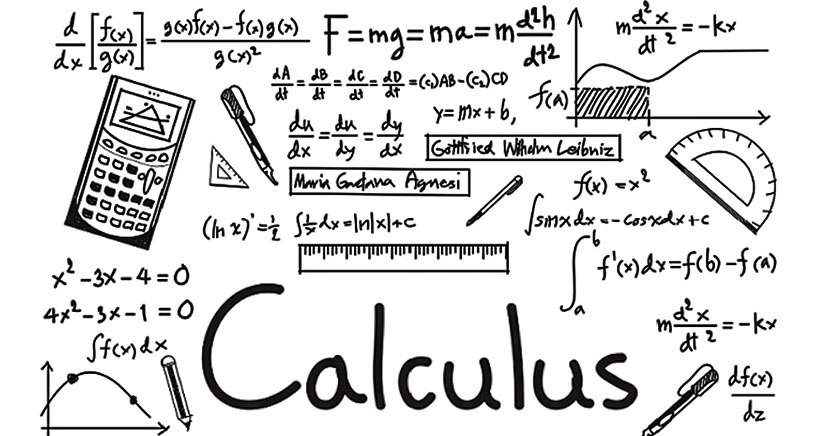## Calculus

0(0 Ratings)

### Calculus

There are many branches of mathematics that man is aware of. Often known as the “language of numbers” for many individuals, it means many things. Some can recognize it as a useful tool that is a key to rolling out civilizations. But it is found by some as an academic inconvenience that only serves lower grade transcripts.

Nevertheless, what can’t be denied is that mathematics is here to stay and it is really a part of our lives, right down to the most basic stuff.

Calculus is one of the foremost branches of mathematics. The systematic study of calculus by well-known scientists and mathematicians such as Isaac Newton and Gottfried Leibniz began in the 17th century, it may have been in use as early as the Greek period, though. It is a mathematical discipline that, to name only a few, primarily deals with functions, limits, derivatives, and integrals. In the history of mathematics, this discipline has a special legacy.

In order to arrive at an optimal solution, it is used to construct mathematical models. In physics, for instance, calculus is used for many of its principles. Motion, electricity, heat, light, harmonics, acoustics, astronomy, and dynamics are among the physical concepts that utilize calculus concepts.

### What jobs do calculus use?

Although there may be several more jobs that might gain from calculus expertise, there are many jobs that involve calculation to make different kinds of estimates, forecasts, and models on a regular basis. Here, in three separate fields (engineering, computer science, and business), we discuss only a few of the available calculus careers and their job descriptions.

### A Few Of Them Are Listed Below

Mathematician for Math

Developer of Apps

Post-secondary educator

Economist

Chemical engineering

Research Analyst in Operations

Electrical engineering

Environmental engineering

#### Get a Free Trial.

We are waiting for you , Get a free trial class today.